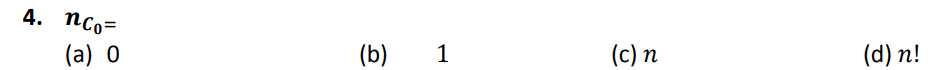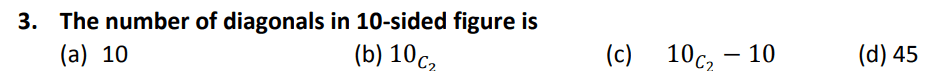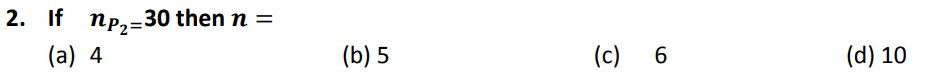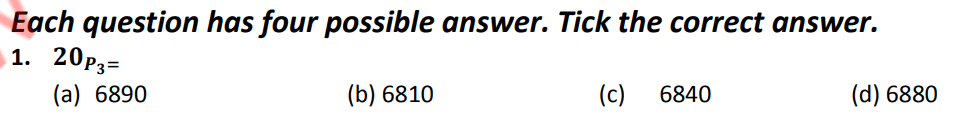# FSC Mathematics MCQs Chapter 7

FSC Math MCQs NUST entry test 2023 NET contains most of the Mathematics MCQs. FSC Part 1 Math book MCQs. HSSC Mathematics questions MCQs. Chapterwise mathematics MCQs for first year 11 class MCQs.

206
Created on By Ali Durrani

Math Ch 7 Permutation, Combination and Probability

1 / 31

Non occurrence of an event E is denoted by:

2 / 31

If an event always occurs , then it is called:

3 / 31

Sample space for tossing a coin is:

4 / 31

. The number of ways for sitting 4 persons in a train on a straight sofa is:

5 / 31

A die is rolled , the probability of getting 3 or 5 is:

6 / 31

16. Four persons wants to sit in a circular sofa, the total ways are:

7 / 31

A card is drawn from a deck of 52 playing cards. The probability of card that it is an ace card is:

8 / 31

Probability of non-occurrence of an event E is equal to :

9 / 31

How many signals can be given by 5 flags of different colors , using 3 at a time

10 / 3111 / 3112 / 31

The number of diagonals in 10-sided figure is

13 / 31

A coin is tossed 5 times , then 𝒏(𝑺) is equal to:

14 / 31

A die is rolled , the probability of getting 3 or 5 is:

15 / 31

Let 𝑺 = {𝟏, 𝟐, 𝟑, … , 𝟏𝟎} the probability that a number is divided by 4 is :

16 / 31

Four persons wants to sit in a circular sofa, the total ways are:

17 / 3118 / 3119 / 3120 / 3121 / 3122 / 3123 / 3124 / 31

How many signals can be given by 5 flags of different colors , using 3 at a time

25 / 3126 / 31

If 𝒏 is negative then 𝒏! is

27 / 3128 / 3129 / 3130 / 3131 / 31